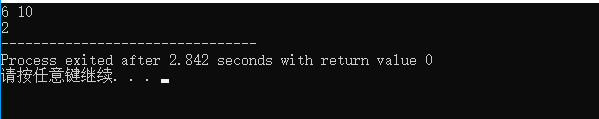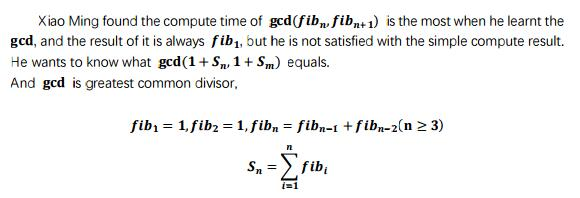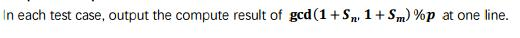gcd_gcdasyncsocket - CSDN
• 求两个数最大公约数，利用欧几里德算法，辗转相除法。详细内容看资料，留作备份。
•GCD
• __gcd-最大公约数 最大公约数（greatest common divisor，简写为gcd；或highest common factor，简写为hcf） __gcd(x,y)是algorithm库中的函数 #include #include using namespace std; int n,m; int main() { ...
__gcd-最大公约数

最大公约数（greatest common divisor，简写为gcd；或highest common factor，简写为hcf）
__gcd(x,y)是algorithm库中的函数

#include<cstdio>
#include<algorithm>
using namespace std;
int n,m;
int main()
{
scanf("%d %d",&n,&m);
int k=__gcd(n,m);//最大公约数
printf("%d",k);
return 0;
}展开全文• 简单的描述就是，记gcd(a,b)表示非负整数a,b的最大公因数，那么：gcd(a,b)=gcd(b,a%b)或者gcd(a,0)=gcd(0,a)=a。   写成程序很简单，不管是用递归还是循环：   int gcd(int a,int b) {  if
历史上第一个称得上算法的好像就是这个欧几里得算法，其实就是地球人都知道的辗转相除，不要小看她，她是很美的。

简单的描述就是，记gcd(a,b)表示非负整数a,b的最大公因数，那么：gcd(a,b)=gcd(b,a%b)或者gcd(a,0)=gcd(0,a)=a。

写成程序很简单，不管是用递归还是循环：

int gcd(int a,int b)
{
if(a==0)
return b;
if(b==0)
return a;
return gcd(b,a%b);
}

设有两个数num1和num2，假设num1比较大。令余数r = num1 % num2。
当r == 0时，即num1可以被num2整除，显然num2就是这两个数的最大公约数。
当r != 0时，令num1 = num2（除数变被除数），num2 = r（余数变除数），再做 r = num1 % num2。递归，直到r == 0。
以上数学原理可以用具体的两个数做一下分析，这样容易理解。

代码实现（求最大公约数）：

不仅算法形式简单，而且效率很高，我不知道具体是多少复杂度的，我只知道效率很高；）

前天看RSA算法，是非对称加密的标准算法，其实算法很简单：
找到两个素数p,q，再找一个数r，使gcd(r,(p-1)(q-1))=1，也就是说互素，然后再找一个数m，使rm=1(mod (p-1)(q-1))，然后再作乘法n=pq，然后把pq丢掉，最好是让任何人都不知道，包括自己（免得说梦话的时候被人听到），然后手里拿到r,m,n，r就是Private Key，只有你知道，而m,n就是Public Key。设信息为a,加密过程：a^r=b (mod n)，b就是密文，解密过程：b^m=a(mod n)，反过来用m加密，用r解密是一样的。

书上说由gcd(r,(p-1)(q-1))=1到求m，使rm=1(mod (p-1)(q-1))是很容易的，就用辗转相除，我想了好久才想到一个方法。

问题：如果gcd(a,b)=1，求x，使ax=1(mod b)
由gcd(a,b)=1可知x是一定存在的，因为前式等同于：存在这样的x,y使ax+by=1，把by拿过去就是ax=-yb+1，即ax=1(mod b)

我令r0=a,r1=b，开始辗转相除
r0=q2r1+r2
r1=q3r2+r3
……
r(s-1)=q(s+1)r(s)+r(s+1)，r(s+1)=1（一定存在着某个r(s+1)=1）

再把余数专门写到一边：
r0=a
r1=b
r2=r0-q2r1
r3=r1-q3r2
……
1=r(s+1)=r(s-1)-q(s+1)r(s)

后面的式子是关于前面的式子的多项式，而最开始是a和b，由最后一个式子就可以证明一定存在1=ax+by，它们都是关于a,b的一次多项式，那如何求x?把前面的式子代到后面，一个一个代，但是你会发现很复杂，不太容易求，于是我想到的就是同样的办法迭代。

设经过从前面的式子的代换，可以得到r(n)=x(n)a+y(n)b，那么有
r(n+1)=r(n-1)-q(n+1)r(n)
=x(n-1)a+y(n-1)b-q(n+1)(x(n)a+y(n)b)
=(x(n-1)-q(n+1)x(n))a+(...)b

于是得到x(n)的迭代式：x(n)=x(n-2)-q(n)x(n-1)，同时有初值x0=1,x1=0，而q(n)=[r(n-2)/r(n-1)]，于是x(n)是确定可求的。一个小小的问题是这样求出的x可能是负数，很简单，在mod b的情况下只需要加上b就行了。

代码：
#include<assert.h>
#include<iostream.h>

int euc(int r1,int r2,int x1,int x2)
{
if(r2==1)
return x2;
if(r2==0)
return 0;
return euc(r2,r1%r2,x2,x1-r1/r2*x2);
}

int euclid(int a,int b)
{
assert(a>0&&b>0);
int x=euc(b,a%b,0,1);
if(x<0)
x+=b;
return x;
}

int main(void)
{
int a,b,x;
cin>>a>>b;
x=euclid(a,b);
if(x==0)
cout<<"gcd(a,b)!=1"<<endl;
else
cout<<"x="<<x<<endl;
return 0;
}

算法的性能和Euclid算法一致，但离RSA还很远。RSA的安全性建立在n=pq的大素数的分解上，老师说一般选几百bit。于是上面这些全部需要改写，需要一个大数运算库，支持四则运算，这都不算什么，Euclid算法还是会很快收敛，关键是在加/解密时的运算，运算量大，所以RSA一般用于加密很小的数据，比如DES的密钥。

另一个方面，我觉得在大数中挑选p,q，以及找r比较困难，不知道用的什么算法，如果真不好算，可以做一个大素数表，每次从中挑几个，表做大一些安全性也不低。


展开全文算法 加密 解密
• 在翻别人的题解的时候偶然发现了这个函数，然后就去查了查，但是相关内容不多，__gcd(x,y);好像是GNU的内部函数，不是一个标准库里的函数，平时写题直接用这个函数挺方便的，int、long long类型都可以，需要注意的是...


在翻别人的题解的时候偶然发现了这个函数，然后就去查了查，但是相关内容不多，__gcd(x,y);好像是GNU的内部函数，不是一个标准库里的函数，平时写题直接用这个函数挺方便的，int、long long类型都可以，需要注意的是两个类型必须要相同，还有就是不能用浮点型，当然也可以手写gcd函数，它头文件是algorithm。

#include <iostream>
#include <algorithm>
using namespace std;
int a,b;

int main()
{
cin>>a>>b;
cout<<__gcd(a,b)<<endl;
return 0;
}



展开全文• ## GCD

2018-11-10 17:20:16
题目描述 输入  The first line is an positive integer T . (1&lt;=T&lt;= 10^3) indicates the number of test cases. In the next T lines, there are three positive integer n, m, p...=10^9) ...
题目描述输入

The first line is an positive integer  T . (1<=T<= 10^3) indicates the number of test cases. In the next T lines, there are three positive integer n, m, p (1<= n,m,p<=10^9) at each line.

输出样例输入

1
1 2 3



样例输出

1

先把所有的1+Sn算出来，你会发现1+Sn=A（n+2）；

再双重循环算出前面几个gcd（1+Sn，1+Sm）%p，结果是A【gsd（n+2,m+2）】%p；

直接循环解决就行了

#include<stdio.h>
long long gcd(long long a,long long b)
{
long long t;
while(b)
{
t=a%b;
a=b;
b=t;
}
return a;
}
int main()
{
long long t,m,n,p,i,sum,sum1,sum2,ans;
while(scanf("%lld",&t)!=EOF)
{
while(t--)
{
scanf("%lld%lld%lld",&n,&m,&p);
if(n>m)
ans=gcd(n+2,m+2);
else
ans=gcd(m+2,n+2);
if(ans==0||ans==1||ans==2)
sum=1;
sum1=1;
sum2=1;
for(i=2;i<ans;i++)
{
sum=sum1+sum2;
sum=sum%p;
sum1=sum2;
sum2=sum;
}
printf("%lld\n",sum);
}
}
return 0;
}


展开全文• ## Gcd

2019-07-17 18:11:56
Gcd Time Limit: 2000/1000 MS (Java/Others) Memory Limit: 524288/524288 K (Java/Others) Total Submission(s): 821 Accepted Submission(s): 275 Problem Description wls 有一个整数 n，他想将 1 − n 这 n...
• 摘自百度百科描述: 辗转相除法， 又名欧几里德算法（Euclidean algorithm），是求最大公约数的一种方法。它的具体做法是：用较小数除较大数，再用出现的余数（第一余数）去除除数，再用出现的余数（第二余数）去除...gcd 辗转相除法
• _gcd(x,y); int、long long类型都可以，需要注意的是两个类型必须要相同，还有不能用浮点型，当然手写gcd函数也是可以的，它头文件是algorithm。 #include<bits/stdc++.h> #include <iostream> #...
• 看了快半个月的GCD源码，只能说太变态了。 先来吐槽一下：一个函数，调用栈都是十几层…… 为了效率，代码使用了纯C语言，但是为了模拟面向对象中的继承，虚函数等，定义了一层层的宏定义，看一个struct的定义要绕...GCD Queue
• 介绍：Grand Central Dispatch 简称（GCD）是苹果公司开发的技术，以优化的应用程序支持多核心处理器和其他的对称多处理系统的系统。这建立在任务并行执行的线程池模式的基础上的。它首次发布在Mac OS X 10.6 ，iOS ...
• ## gcd

2019-07-16 19:09:08
gcd gcd(a,b)=gcd(b,a mod b) 边界条件:gcd(a,0)=a gcd(a,b) * lcm(a,b)=a*b 于是求lcm: a/gcd(a,b)*b; 先除保证中间结果不会溢出 //递归写法 int gcd(int a,int b) { return b==0?a:gcd(b,a%b); } //求a%b余数 ...gcd
• 1.欧几里得算法 欧几里得是求最大公约数的...gcd(a,b)=gcd(b,a)=gcd(-a,b)=gcd(|a|,|b|) gcd(a,b)=gcd(b,a mod b) 证明：a可以表示成a = kb + r，则r = a mod b 假设d是a,b的一个公约数， 则有 d | a , d...gcd 算法
• 在iOS开发中经常会用到GCD，如果你在求职过程中GCD的使用也是面试官必问的，那么今天就来说说GCD的有关内容，不喜勿喷。 一、GCD的概念 1.GCD全称是Grand Central Dispatch，可译为“CPU的中枢调度器”，是C语言，...iOS开发 gcd 同步异步
• Gcd最大公约数 python def gcd(a, b): if a % b == 0: return b else: return gcd(b, a % b) C++ 简单原始版本： int gcd(int a,int b){ return a%b == 0 ? b : gcd(b,a%b); } 简化一点 int gcd(int a,int b)...
• Neko does Maths //gcd(a,b)=gcd(b−a,a) 题意：给a和b，求a和b加上一个k之后的最小lcm 官方题解： gcd(a+k,b+k)=gcd(b−a,a+k) 因为gcd(a,b)=gcd(b%a,a) 然后枚举因子，枚举因子日常枚举根号(b-a)即可。 ...gcd
• 欧几里德算法(gcd)又称辗转相除法，用于计算两个整数a,b的最大公约数。 基本思路：设a=qb+r，其中a，b，q，r都是整数，则gcd(a,b)=gcd(b,r)，即gcd(a,b)=gcd(b,a%b)。 代码（python）：def gcd(a,b): if b==0: ...算法 gcd
• //求解ax+my=1 int exGCD(int a,int b,int &amp;x,int &amp;y) { if(b==0) { x=1; y=0; return a; } int g=exGCD(b,a%b,x,y); int tmp=x; x=y;...int gcd(int a,int b) { if...最大公因数
• 1.5 用GCD执行与UI相关的任务 目的：为了并发你使用了GCD并且想知道与UI相关的APIs一起工作的最佳办法。 讨论：UI相关的任务必须在主线程中执行，所以主队列是在GCD中执行UI任务的唯一候选对象。我们可以使用...iOS GCD
• 在我们实际的开发中，我们往往有这样的需求，就是需要先执行完线程1，再执行...代码我会放在github ，的GCD03中。 （1）同样也需要导入GCD源码。然后在ViewController中实现如下： #import "ViewController.h" #impo线程
...